# 9.3 - Identifying Outliers (Unusual Y Values)

Previously in Lesson 4 we mentioned two measures that we use to help identify outliers. They are:

• Residuals
• Standardized Residuals

We briefly review these measures here. However, this time, we add a little more detail.

### Residuals

As you know, ordinary residuals are defined for each observation, i = 1, ..., n as the difference between the observed and predicted responses:

$e_i=y_i-\hat{y}_i$

For example, consider the following very small (contrived) data set containing n = 4 data points (x, y).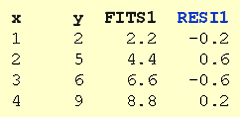The column labeled "FITS1" contains the predicted responses, while the column labeled "RESI1" contains the ordinary residuals. As you can see, the first residual (-0.2) is obtained by subtracting 2.2 from 2; the second residual (0.6) is obtained by subtracting 4.4 from 5; and so on.

As you know, the major problem with ordinary residuals is that their magnitude depends on the units of measurement, thereby making it difficult to use the residuals as a way of detecting unusual y values. We can eliminate the units of measurement by dividing the residuals by an estimate of their standard deviation, thereby obtaining what are known as standardized residuals.

### Standardized residuals

Standardized residuals (sometimes referred to as "internally studentized residuals") are defined for each observation, i = 1, ..., n as an ordinary residual divided by an estimate of its standard deviation:

$r_{i}=\frac{e_{i}}{s(e_{i})}=\frac{e_{i}}{\sqrt{MSE(1-h_{ii})}}$

Here, we see that the standardized residual for a given data point depends not only on the ordinary residual, but also the size of the mean square error (MSE) and the leverage hii.

For example, consider again the (contrived) data set containing n = 4 data points (x, y):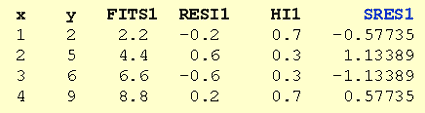The column labeled "FITS1" contains the predicted responses, the column labeled "RESI1" contains the ordinary residuals, the column labeled "HI1" contains the leverages hii, and the column labeled "SRES1" contains the standardized residuals. The value of MSE is 0.40. Therefore, the first standardized residual (-0.57735) is obtained by:

$r_{1}=\frac{-0.2}{\sqrt{0.4(1-0.7)}}=-0.57735$

and the second standardized residual is obtained by:

$r_{2}=\frac{0.6}{\sqrt{0.4(1-0.3)}}=1.13389$

and so on.

The good thing about standardized residuals is that they quantify how large the residuals are in standard deviation units, and therefore can be easily used to identify outliers:

• An observation with a standardized residual that is larger than 3 (in absolute value) is deemed by some to be an outlier. [It is technically more correct to reserve the term "outlier" for an observation with a studentized residual that is larger than 3 in absolute value—we consider studentized residuals in the next section.]
• Some statistical software flags any observation with a standardized residual that is larger than 2 (in absolute value).

Using a cutoff of 2 may be a little conservative, but perhaps it is better to be safe than sorry. The key here is not to take the cutoffs of either 2 or 3 too literally. Instead, treat them simply as red warning flags to investigate the data points further.

### Example #2 (again)

Let's take another look at the following data set (influence2.txt)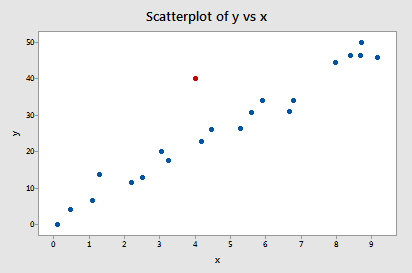In our previous look at this data set, we considered the red data point an outlier, because it does not follow the general trend of the rest of the data. Let's see what the standardized residual of the red data point suggests: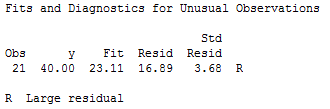Indeed, its standardized residual (3.68) leads this software to flag the data point as being an observation with a "Large residual."

### Why should we care about outliers?

We sure spend an awful lot of time worrying about outliers. But, why should we? What impact does their existence have on our regression analyses? One easy way to learn the answer to this question is to analyze a data set twice—once with and once without the outlier—and to observe differences in the results.

Let's try doing that to our Example #2 data set. If we regress y on x using the data set without the outlier, we obtain: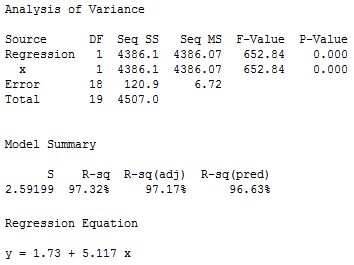And if we regress y on x using the full data set with the outlier, we obtain: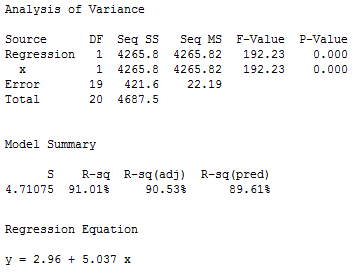What aspect of the regression analysis changes substantially because of the existence of the outlier? Did you notice that the mean square error MSE is substantially inflated from 6.72 to 22.19 by the presence of the outlier? Recalling that MSE appears in all of our confidence and prediction interval formulas, the inflated size of MSE would thereby cause a detrimental increase in the width of all of our confidence and prediction  intervals. However, as noted in Section 9.1, the predicted responses, estimated slope coefficients, and hypothesis test results are not affected by the inclusion of the outlier. Therefore, the outlier in this case is not deemed influential (except with respect to MSE).